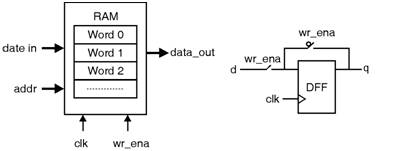Home > VHDL > Shift Registers > RAM

Random Access Memory

Here, the implementation of RAM (random access memory) is shown. The system has a data input bus (data_in), a data output bus (data_out), an address bus (addr), along with clock (clk) and write enable (wr_ena) pins. When wr_ena is given, at the next rising edge of clk the vector present at data_in stored in the position given by addr. The capacity chosen for the RAM is 16 words of length 8 bits each. Notice that the code is totally generic. Simulation results from the circuit synthesizad with the VHDL code are shown below.LIBRARY ieee;

USE ieee.std_logic_1164.all;

ENTITY ram IS

GENERIC ( bits: INTEGER := 8; -- # of bits per word

words: INTEGER := 16); -- # of words in the memory

PORT ( wr_ena, clk: IN STD_LOGIC;

addr: IN INTEGER RANGE 0 TO words-1;

data_in: IN STD_LOGIC_VECTOR (bits-1 DOWNTO 0);

data_out: OUT STD_LOGIC_VECTOR (bits-1 DOWNTO 0));

END ram;

ARCHITECTURE ram OF ram IS

TYPE vector_array IS ARRAY (0 TO words-1) OF

STD_LOGIC_VECTOR (bits-1 DOWNTO 0);

SIGNAL memory: vector_array;

BEGIN

PROCESS (clk, wr_ena)

BEGIN

IF (wr_ena='1') THEN

IF (clk'EVENT AND clk='1') THEN